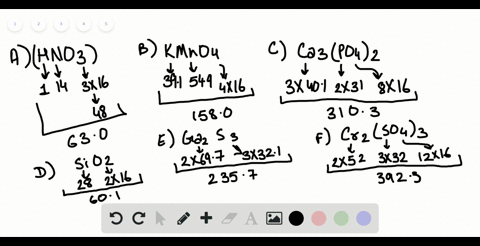Sign up for our free STEM online summer camps starting June 1st!View Summer Courses### Determine the formula weights of each of the foll…

00:28Auburn University Main Campus

Need more help? Fill out this quick form to get professional live tutoring.

Get live tutoring
Problem 22

Balance the following equations and indicate whether they are combination, decomposition, or combustion reactions:
$$\begin{array}{l}{\text { (a) } \mathrm{PbCO}_{3}(s) \longrightarrow \mathrm{PbO}(s)+\mathrm{CO}_{2}(g)} \\ {\text { (b) } \mathrm{C}_{2} \mathrm{H}_{4}(g)+\mathrm{O}_{2}(g) \longrightarrow \mathrm{CO}_{2}(g)+\mathrm{H}_{2} \mathrm{O}(g)} \\ {\text { (c) } \mathrm{Mg}(s)+\mathrm{N}_{2}(g) \longrightarrow \mathrm{Mg}_{3} \mathrm{N}_{2}(s)} \\ {\text { (d) } \mathrm{C}_{7} \mathrm{H}_{8} \mathrm{O}_{2}(l)+\mathrm{O}_{2}(g) \longrightarrow \mathrm{CO}_{2}(g)+\mathrm{H}_{2} \mathrm{O}(g)} \\ \text { (e) } \mathrm{Al}(s)+\mathrm{Cl}_{2}(g) \longrightarrow \mathrm{AlCl}_{3}(s) \end{array}$$

c) $3 \mathrm{Mg}+\mathrm{N} 2 \ldots \mathrm{Mg3N} 2$
Combination reaction

## Discussion

You must be signed in to discuss.